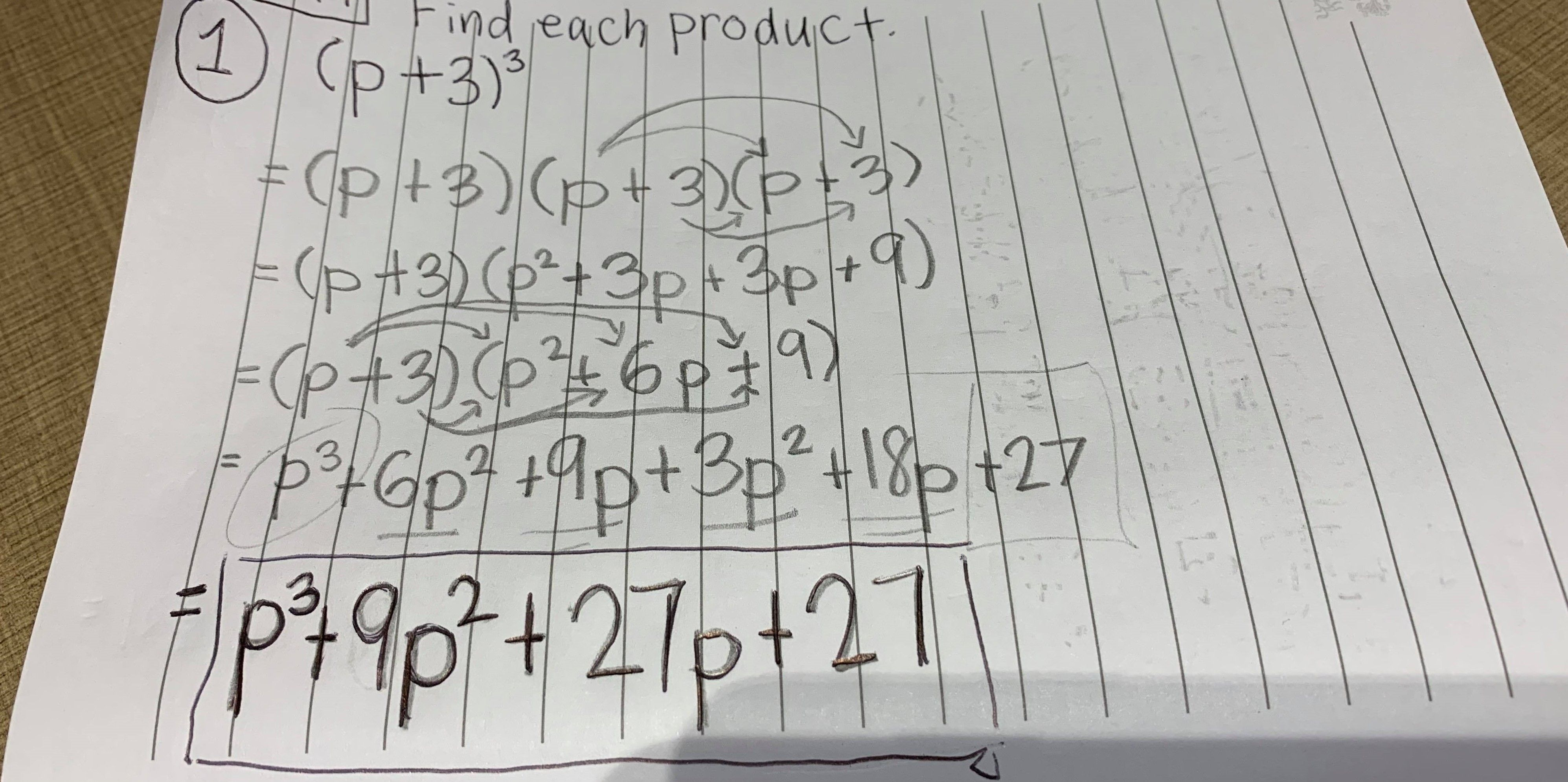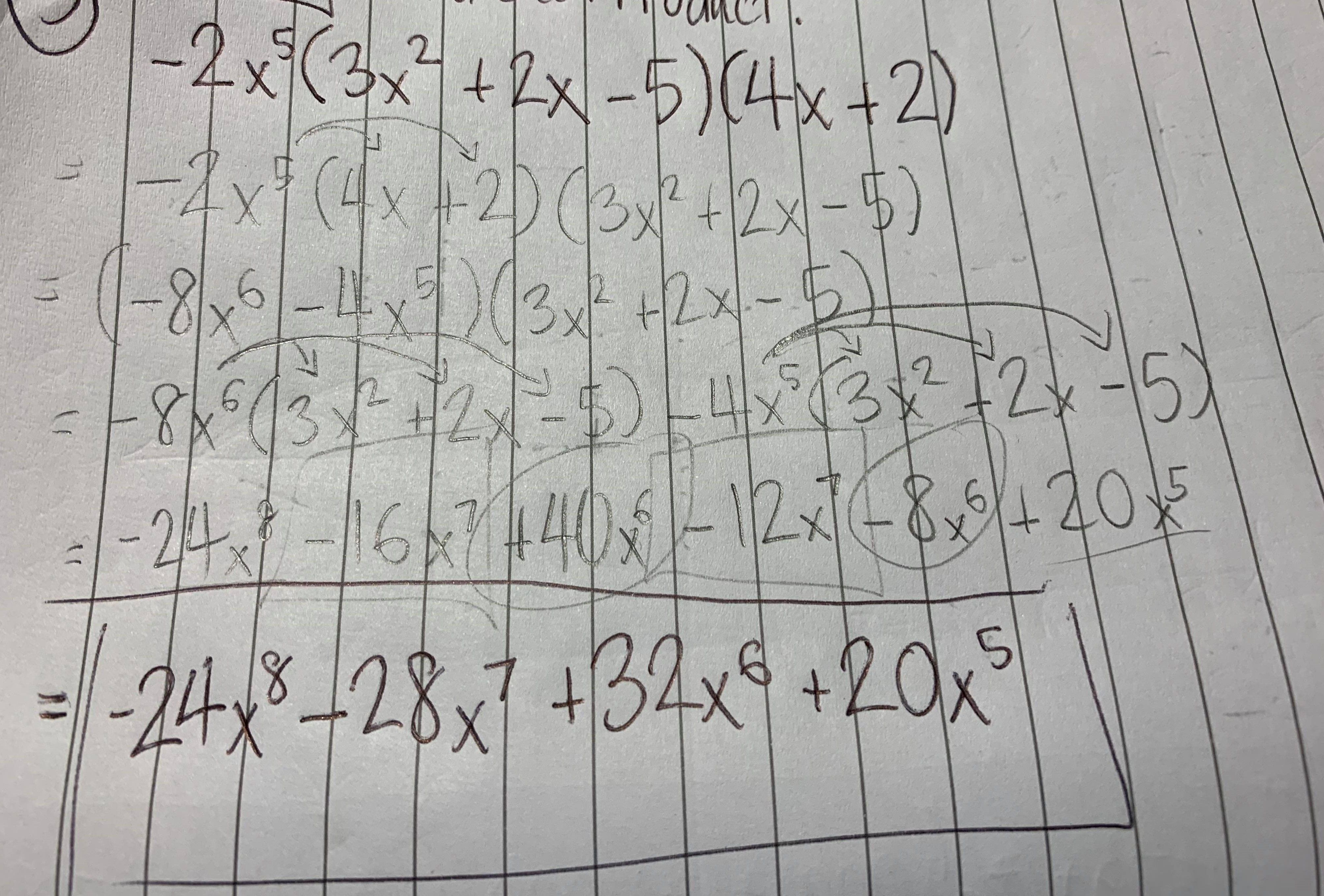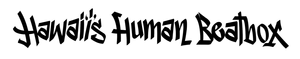## Math SpeaksEver had a conversation with someone who asks you the same question even after you've already responded to him or her your answer? I will use mathematics to as an analogy to share this scenario.

Person asks: What is the answer to (p + 3)³ (translation: what is the cubed evaluation of the binomial p plus three)?

You: You want to know the answer to (p + 3)³? Well here it is......
(p + 3)³
= (p + 3)(p + 3)(p + 3)
= (p + 3)(p² + 6p + 9)
= p(p² + 6p + 9) + 3(p² + 6p + 9)
= p³ + 6p² + 9p + 3p² + 18p + 27
= p³ + 6p² + 3p² + 9p + 18p + 27
= p³ + 9p² + 27p + 27 (final answer)
That is the answer to (p + 3)³.Person (months later): Wait, you typed all of that beautifully, but I don't get it, what is the answer to (p + 3)³? You did not answer me.

You: I answered your question what the answer to (p + 3)³ is. Our conversation was done months ago.

Person: What is the answer to (p + 3)³?

You: Here it is, the answer to (p + 3)³......
(p + 3)³
= (p + 3)(p + 3)(p + 3)
= (p + 3)(p² + 6p + 9)
= p(p² + 6p + 9) + 3(p² + 6p + 9)
= p³ + 6p² + 9p + 3p² + 18p + 27
= p³ + 6p² + 3p² + 9p + 18p + 27
= p³ + 9p² + 27p + 27 (final answer)
That is the answer to (p + 3)³.

Person: What is the answer to (p + 3)³? You did not include the answer.

You: I answered your question. Our conversation is done. Mahalo!

Bonus Math Problem: here is of another math problem that could also be used in this scenario.Math and Beatbox Music Speaks